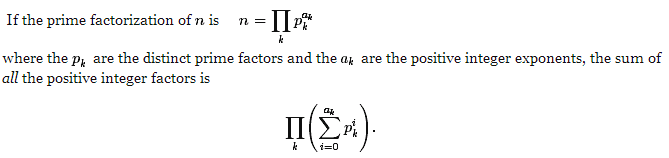# Project Euler

### A Taste of Number Theory

#### Problem 23: Non-abundant Sums

A perfect number is a number for which the sum of its proper divisors is exactly equal to the number. For example, the sum of the proper divisors of 28 would be 1 + 2 + 4 + 7 + 14 = 28, which means that 28 is a perfect number.

A number n is called deficient if the sum of its proper divisors is less than n and it is called abundant if this sum exceeds n.

As 12 is the smallest abundant number, 1 + 2 + 3 + 4 + 6 = 16, the smallest number that can be written as the sum of two abundant numbers is 24. By mathematical analysis, it can be shown that all integers greater than 28123 can be written as the sum of two abundant numbers. However, this upper limit cannot be reduced any further by analysis even though it is known that the greatest number that cannot be expressed as the sum of two abundant numbers is less than this limit.

Find the sum of all the positive integers which cannot be written as the sum of two abundant numbers.

#### The Catch

How to efficiently find the sum of all proper divisors.
How to determine if a number can be written as a sum of 2 abundant numbers.

#### The Light

We can certainly use the implementation in Problem 21 to find the sum of all proper divisors. There is another way, however, that is just as elegant: the Omega function:For example, the sum of all factors of 120 = 23 x 3 x 5 is (1 + 2 + 23 + 23)(1 + 3)(1 + 5) = 15 x 4 x 6 = 360.

To find the sum of all proper divisors, subtract the original number n from the sum found above. Make some modification to the prime factorization in Problem 12 to implement the Omega function.

To determine if a number can be written as the sum of 2 abundant numbers, make a boolean array of size 28,123. Create a list of abundant number up to 28,123 using the modified prime factorization method. Then make 2 for loops: the outer loops from the first abundant number a to the last one in the list and the inner loops from a to the last one in the list. If the sum of the number from these 2 loops is less than or equal to 28,123, then that sum can be written as the sum of 2 abundant numbers. Mark this number in the boolean array. When the two loops terminate, sum up all the numbers that were not marked (i.e numbers that cannot be written as the sum of 2 abundant numbers).

Wolfram Alpha's article on abundant numbers states that the upper bound is actually 20,161, so use that as the upper bound instead of 28,123 (saving almost 8,000 iterations!)

#### The Code

```import java.util.*;

public class Problem23
{
static List primeList = generatePrimes(20161);

public static void main(String[] args)
{
List abundant = new ArrayList();
long sum = 0;

for(int i = 12; i < 20161; i++)
{
if(sumProperDiv(i) > i)
}

boolean[] sumOfAbundant = new boolean[20161 + 1];
for(int i = 0; i < abundant.size(); i++)
{
for(int j = i; j < abundant.size(); j++)
{
if(abundant.get(i) + abundant.get(j) <= 20161)
sumOfAbundant[abundant.get(i) + abundant.get(j)] = true;
else
break;
}
}

for(int i = 0; i <= 20161; i++)
{
if(!sumOfAbundant[i])
sum += i;
}
System.out.println(sum);
}

public static int sumProperDiv(int n)
{
int notProper = n;
int index = 0;
double sum = 1.0;
while(n > 1)
{
int pow = 1;
double subSum = 1.0;
while(n % primeList.get(index) == 0)
{
n /= primeList.get(index);
subSum += Math.pow(primeList.get(index),pow++);
}
sum *= subSum;
index++;
}

return (int)sum - notProper;
}

public static List generatePrimes(int max)
{
boolean[] isComposite = new boolean[max + 1];
for (int i = 2; i * i <= max; i++)
{
if (!isComposite [i])
for (int j = i; i * j <= max; j++)
{
isComposite [i*j] = true;
}
}
ArrayList list = new ArrayList();

for (int i = 2; i <= max; i++)
{
if (!isComposite [i])